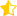Learn from home

The teachersChapters

### Probability Axioms

1.The probability is positive and less than or equal to 1.

0 ≤ p(A) ≤ 1

2. The probability of the sure event is 1.

p(S) = 1

3.If A and B are mutually exclusive, then:

p(A B) = p(A) + p(B)

## 1

The sum of the probabilities of an event and its complementary is 1, so the probability of the complementary event is:## 2

The probability of an impossible event is zero.## 3

The probability of the union of two events is the sum of their probabilities minus the probability of their intersection.## 4

If an event is a subset of another event, its probability is less than or equal to it.## 5

If A1, A2, ..., Ak are mutually exclusive between them, then:## 6

If the sample space S is finite and an event is S = {x1, x2, ..., xn} then:For example, the probability of obtaining an even number, when rolling a die, is:

P(even) = P(2) + P(4) + P(6)

Did you like the article?(3 votes, average: 4.67 out of 5)Loading...

Emma

I am passionate about travelling and currently live and work in Paris. I like to spend my time reading, gardening, running, learning languages and exploring new places.

Did you like
this resource?

Bravo!